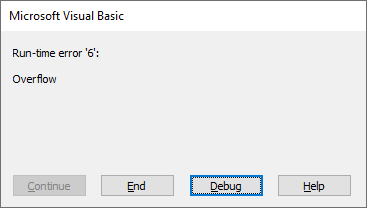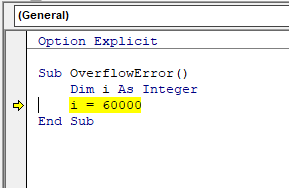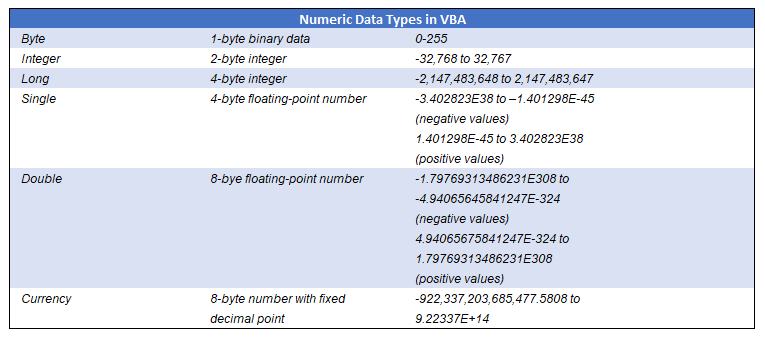# VBA Runtime Error 6Run-time error 6 in VBA is the Overflow error. This means that a variable has been declared as one numeric data type, and then populated with a number that is outside the parameters of that data type.

Consider the following code:

``````Sub OverflowError()
Dim i As Integer
i = 600000
End Sub``````

We are declaring i as an Integer variable. An integer variable can hold the values of ‑32,768 to 32,768.  We are trying to assign the value of 600,000, which is outside the allowed range and therefore the error will occur.

If we click on Debug when this error occurs, the error line will appear in yellow.To solve this error, make sure you declare the variable with the correct numeric data types to hold the required data:In this case use the Long Variable type.

``````Sub OverflowError_Corrected()
Dim i As Long
i = 600000
End Sub``````

We recommend always using the Long variable type instead of the Integer variable type. The only advantage of the Integer variable type is less memory is required. However, this is mostly irrelevant for coding running from VBA on modern computers.